4.4. Symbol Tables

# 4.4 Symbol Tables

A symbol table is a data type that we use to associate values with keys. Clients can store (put) an entry into the symbol table by specifying a key-value pair and then can retrieve (get) the value corresponding to a particular key from the symbol table.

In this chapter we consider a basic API for the symbol-table data type. Our API adds to the put and get operations the abilities to test whether any value has been associated with a given key (contains) and to iterate over the keys. We also consider an extension to the API for the case where keys are comparable, which admits a number of useful operations.

We also consider two classic implementations. The first uses an operation known as hashing, which transforms keys into array indices that we can use to access values. The second is based on a data structure known as the binary search tree (BST).

## API

A symbol table is a collection of key-value pairs — every symbol-table entry associates a value with a key, as follows: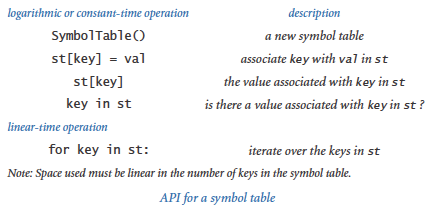The API is consistent with the API for Python's built-in `dict` data type, which we consider later in this section. The API already reflects several design decisions, which we now enumerate.

### Associative arrays.

We overload the `[]` operator for the two basic operations put and get. In client code this means that we can think of a symbol table as an associative array, where we can use standard array syntax with any type of data inside the square brackets instead of an integer between 0 and the length, as for an array. Thus, we can associate a codon with an amino acid name with client code like

 ```amino['TTA'] = 'Leucine' ```

and we can later access the name associated with a given codon with client code like

 ```stdio.writeln(amino['TTA']) ```

That is, an associative array reference is a get operation, unless it is on the left side of an assignment statement, when it is a put operation. We can support these operations by implementing the special methods `__getitem__()` and `__setitem__()`.

### Replace-the-old-value policy.

If a value is to be associated with a key that already has an associated value, we adopt the convention that the new value replaces the old one (just as with an array assignment statement). Again, this is what one would expect from the associative-array abstraction. The `key in st` operation, supported by the special method `__contains__()`, gives the client the flexibility to avoid doing so, if desired.

The call `st[key]` raises a `KeyError` if no value has been associated with key in the table. An alternative design would be to return `None` in such cases.

### None keys and values.

Clients may use `None` as a key or value, though they typically do not do so. An alternative design would be to disallow either `None` keys and/or values.

### Iterable.

To support the `for key in st`: construct, Python's convention is that we need to implement a special method `__iter__()` that returns an iterator, a special data type that includes methods that are called at the beginning and for each iteration of the `for` loop. We consider Python's mechanism for iteration at the end of this section.

### Remove.

Our basic API does not include a method for removing keys from the symbol table. Some applications do require such a method, and Python provides the special syntax `del st[key]` that can be supported by implementing the special method `__delitem__()`. We leave implementations as exercises or for a more advanced course in algorithms.

### Immutable keys.

We assume the keys do not change their value while in the symbol table. The simplest and most commonly used types of keys (integers, floats, and strings) are immutable. If you think about it, you will see that is a very reasonable assumption! If a client changes a key, how could the symbol table implementation keep track of that fact?

### Variations.

Computer scientists have identified numerous other useful operations on symbol tables, and APIs based on various subsets of them have been widely studied. We consider several of these operations throughout this section, and particularly in the exercises at the end.

### Comparable keys.

In many applications, the keys may be integers, floats, strings, or other data types of data that have a natural order. In Python, as discussed in Section'3.3, we expect such keys to be comparable. Symbol tables with comparable keys are important for two reasons. First, we can take advantage of key ordering to develop implementations of put and get that can guarantee the performance specifications in the API. Second, a whole host of new operations come to mind (and can be supported) with comparable keys. A client might want the smallest key, or the largest, or the median, or to iterate over the keys in sorted order. Full coverage of this topic is more appropriate for a book on algorithms and data structures, but we examine a typical client and an implementation of such a data type later in this section. This is a partial API: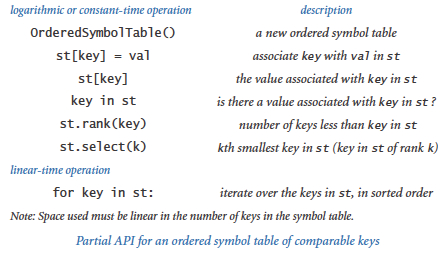## Symbol Table Clients

We start with two prototypical examples, each of which arises in a large number of important and familiar practical applications.

### Dictionary lookup.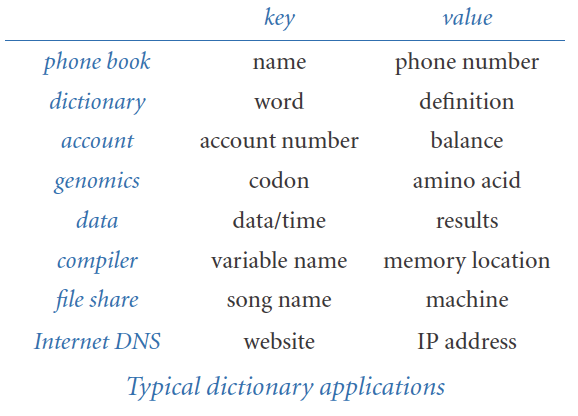The most basic kind of symbol-table client builds a symbol table with successive put operations to support get requests. The program lookup.py builds a set of key-value pairs from a file of comma-separated values as specified on the command line and then writes values corresponding to the keys read from standard input. The command-line arguments are the file name and two integers, one specifying the field to serve as the key and the other specifying the field to serve as the value.

This booksite provides numerous comma-separated-value (.csv) files that you can use as input to lookup.py, including amino.csv (codon-to-amino-acid encodings), djia.csv (opening price, volume, and closing price of the stock market average, for every day in its history), elements.csv (periodic table of elements), ip.csv (a selection of entries from the DNS database), ip-by-country.csv (IP addresses by country), morse.csv (Morse code), and phone-na.csv (telephone area codes). When choosing which field to use as the key, remember that each key must uniquely determine a value. If there are multiple put operations to associate values with a key, the table will remember only the most recent one (think about associative arrays). We will consider next the case where we want to associate multiple values with a key.

### Indexing.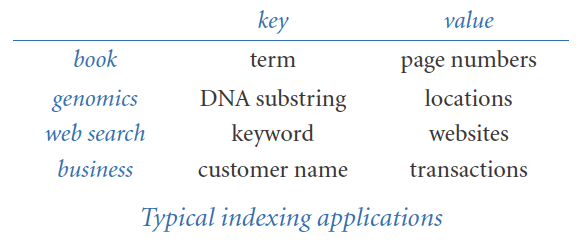The program index.py is a prototypical example of a symbol-table client for comparable keys. It reads in a list of strings from standard input and writes a sorted table of all the different strings along with a list of integers for each string specifying the positions where it appears in the input. In this case, we seem to be associating multiple values with each key, but we actually associating just one: a Python list.

To cut down on the amount of output, index.py takes three command-line arguments: a file name and two integers. The first integer is the minimum string length to include in the symbol table, and the second is the minimum number of occurrences (among the words that appear in the text) to include in the printed index. Try running index.py on the files tale.txt and mobydick.txt.

## Hash Tables

Symbol-table implementations have been heavily studied, many different algorithms and data structures have been invented for this purpose, and modern programming environments (including Python) provide direct support. As usual, knowing how a basic implementation works will help you appreciate, choose among, and more effectively use the advanced ones, or help implement your own version for some specialized situation that you might encounter.

One way to implement a symbol table is as a hash table. A hash table is a data structure in which we divide the keys into small groups that can be quickly searched. The basic idea is simple. We choose a parameter m and divide the keys into m groups, which we expect to be about equal in size. For each group, we keep the keys in a list and use sequential search.

To divide the keys into small groups, we use a function called a hash function that maps every possible key into a hash value — an integer between 0 and m-1. This enables us to model the symbol table as a fixed-length array of lists and use the hash value as an array index to access the desired list. In Python, we can implement both the fixed-length array and the lists using the built-in `list` data type.

Hashing is widely useful, so many programming languages include direct support for it. As we saw in Section 3.3, Python provides the built-in `hash()` function for this purpose, which takes a hashable object as an argument returns an integer hash code. To convert that to a hash value between 0 and m-1, we use the expression

 ```hash(x) % m ```

Recall that an object is hashable if it satisfies the following three properties:

• The object can be compared for equality with other objects.
• Whenever two objects compare as equal, they have the same hash code.
• The object's hash code does not change during its lifetime.

Objects that are not equal may have the same hash code. However, for good performance, we expect the hash function to divide our keys into m groups of roughly equal length.

Implementing an efficient symbol table with hashing is straightforward. For the keys, we maintain an array of m lists, with element `i` containing a Python list of keys whose hash value is `i`. For the values, we maintain a parallel array of m lists, so that when we have located a key, we can access the corresponding value using the same indices. The program hashst.py is a full implementation, using a fixed number of m lists (1024 by default).

The efficiency of hashst.py depends on the value of m and the quality of the hash function. Assuming the hash function reasonably distributes the keys, performance is about m times faster than that for sequential search, at the cost of m extra references and lists. This is a classic space-time tradeoff: the higher the value of m, the more space we use, but the less time we spend.

The primary disadvantage of hash tables is that they do not take advantage of order in the keys and therefore cannot provide the keys in sorted order or support efficient implementations of operations like finding the minimum or maximum. For example, the keys will come out in arbitrary order in index.py, not the sorted order that is called for. Next, we consider a symbol table implementation that can support such operations when keys are comparable, without sacrificing much performance.

## Binary Search Trees

The binary tree is a mathematical abstraction that plays a central role in the efficient organization of information. For symbol-table implementations, we use a special type of binary tree to organize the data and to provide a basis for efficient implementations of the symbol-table put operations and get requests. A binary search tree (BST) associates comparable keys with values, in a structure defined recursively. A BST is one of the following: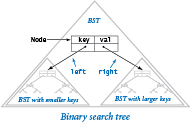• Empty (`None`)
• A node having a key-value pair and two references to BSTs, a left BST with smaller keys and a right BST with larger keys

The keys must be comparable via the < operator.

To implement BSTs, we start with a class for the node abstraction, which has references to a key, a value, and left and right BSTs:

 ```class Node: def __init__(self, key, val): self.key = key self.val = val self.left = None self.right = None ```

This definition is like our definition of nodes for linked lists, except that it has two links, not just one. From the recursive definition of BSTs, we can represent a BST with a variable of type `Node` by ensuring that its value is either `None` or a reference to a `Node` whose left and right instance variables are references to BSTs, and by ensuring that the ordering condition is satisfied (keys in the left BST are smaller than key and keys in the right BST are larger than key).

We often use tree-based terminology when discussing BSTs. We refer to the node at the top as the root of the tree, the BST referenced by its left link as the left subtree, and the BST referenced by its right link as the right subtree. Traditionally, computer scientists draw trees upside down, with the root at the top. Nodes whose links are both null are called leaf nodes. The height of a tree is the maximum number of links on any path from the root node to a leaf node.

Suppose that you want to search for a node with a given key in a BST (or to get a value with a given key in a symbol table). There are two possible outcomes: the search might be successful (we find the key in the BST; in a symbol-table implementation, we return the associated value) or it might be unsuccessful (there is no key in the BST with the given key; in a symbol-table implementation, we raise a run-time error).

A recursive searching algorithm is immediate: Given a BST (a reference to a `Node`), first check whether the tree is empty (the reference is `None`). If so, then terminate the search as unsuccessful (in a symbol-table implementation, raise a run-time error). If the tree is nonempty, check whether the key in the node is equal to the search key. If so, then terminate the search as successful (in a symbol-table implementation, return the value associated with the key). If not, compare the search key with the key in the node. If it is smaller, search (recursively) in the left subtree; if it is greater, search (recursively) in the right subtree.

Suppose that you want to insert a new node into a BST (in a symbol-table implementation, put a new key-value pair into the data structure). The logic is similar to searching for a key, but the implementation is trickier. The key to understanding it is to realize that only one link must be changed to point to the new node, and that link is precisely the link that would be found to be `None` in an unsuccessful search for the key in that node.

If the BST is empty, we create and return a new `Node` containing the key-value pair; if the search key is less than the key at the root, we set the left link to the result of inserting the key-value pair into the left subtree; if the search key is greater, we set the right link to the result of inserting the key-value pair into the right subtree; otherwise, if the search key is equal, we overwrite the existing value with the new value. Resetting the left or right link after the recursive call in this way is usually unnecessary, because the link changes only if the subtree is empty, but it is as easy to set the link as it is to test to avoid setting it.

The program bst.py is a symbol-table implementation based on these two recursive algorithms. As with linkedstack.py and linkedqueue.py (from Section 4.3), we use a private `_Node` class to emphasize that clients of `OrderedSymbolTable` do not need to know any of the details of the binary search tree representation.

## Performance Characteristics of BSTs

The running times of BST algorithms are ultimately dependent on the shape of the trees, and the shape of the trees is dependent on the order in which the keys are inserted.

### Best case.

In the best case, the tree is perfectly balanced (each `Node` has exactly two children that are not `None`, except the nodes at the bottom, which have exactly two children that are `None`), with lg n nodes between the root node and each leaf node. In such a tree, it is easy to see that the cost of an unsuccessful search is logarithmic, because that cost satisfies the same recurrence relation as the cost of binary search (see Section 4.2) so that the cost of every put operation and get request is proportional to lg n or less. You would have to be quite lucky to get a perfectly balanced tree like this by inserting keys one by one in practice, but it is worthwhile to know the best possible performance characteristics.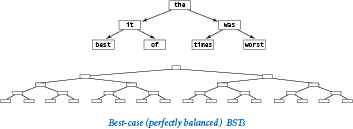### Average case.

If we insert random keys, we might expect the search times to be logarithmic as well, because the first key becomes the root of the tree and should divide the keys roughly in half. Applying the same argument to the subtrees, we expect to get about the same result as for the best case.### Worst case.

In the worst case, each node has exactly one `None` link, so the BST is like a linked list with an extra wasted link, where put operations and get requests take linear time. Unfortunately, this worst case is not rare in practice — it arises, for example, when we insert the keys in order.

Thus, good performance of the basic BST implementation is dependent on the keys being sufficiently similar to random keys that the tree is not likely to contain many long paths. If you are not sure that assumption is justified, do not use a simple BST. Remarkably, there are BST variants that eliminate this worst case and guarantee logarithmic performance per operation, by making all trees nearly perfectly balanced. One popular variant is known as a red-black tree.

## Traversing a BST

Perhaps the most basic tree-processing function is known as tree traversal: given a (reference to) a tree, we want to systematically process every key-value pair in the tree. To process every key in a BST we use this recursive approach: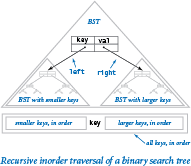• Process every key in the left subtree.
• Process the key at the root.
• Process every key in the right subtree.

This approach is known as inorder tree traversal, to distinguish it from preorder (do the root first) and postorder (do the root last), which arise in other applications. For example, the following method writes the keys in the BST rooted at its argument in key-sorted order:

 ```def inorder(x): if x is None: return inorder(x.left) stdio.writeln(x.key) inorder(x.right) ```

## Iterables

As you learned in Section 1.3 and Section 1.4, you can use a `for` loop to iterate over either integers in a range or elements in an array `a[]`.

 ```for i in range(n): for v in a: stdio.writeln(i) stdio.writeln(v) ```

The `for` loop is not just for integer ranges and arrays — you can use it with any iterable object. An iterable object is an object that is capable of returning its items one at a time. All of Python's sequence types — including `list`, `tuple`, `dict`, `set`, and `str` — are iterable, as is the object returned by the built-in `range()` function.

Now, our goal is to make `SymbolTable` iterable, so that we can use a `for` loop to iterate over its keys (and use indexing to get the corresponding values):

 ```st = SymbolTable() ... for key in st: stdio.writeln(str(key) + ' ' + str(st[key])) ```

To make a user-defined date type iterable, you must implement the special method `__iter__()`, in support of the built-in function `iter()`. The `iter()` function creates and returns an iterator, which is a data type that includes a special method `__next__()` that Python calls at the beginning of each iteration of a `for` loop.

While this appears complicated, we can use a shortcut based on the fact that Python lists are iterable: if `a` is a Python list, then `iter(a)` returns an iterator over its items. So we can make our hash table and binary search tree implementations iterable by collecting the keys in a Python list and returning an iterator for that list.

To make hashst.py iterable, we accumulate all keys into a Python list, and then return an iterator over the list; the `__iter__()` method in hashst.py does precisely that. To make bst.py iterable, we modify the recursive `inorder()` method shown above to collect the keys in a Python list instead of writing them. Then we can return an iterator for that list. The `_inorder()` and `__iter__()` methods in bst.py use that approach.

The flexibility of BSTs and the ability to compare keys enables the implementation of many useful operations beyond those that can be supported efficiently in hash tables. For example, with a BST we can efficiently find the minimum or maximum key, find all keys within a specified range, and find the kth smallest key. We leave implementations of these operations for exercises and leave further study of their performance characteristics and applications for a course in algorithms and data structures.

## Dictionary Data Type

Now that you understand how a symbol table works, you are ready to use Python's industrial-strength version. The built-in `dict` data type follows the same basic API as `SymbolTable`, but with a richer set of operations, including deletion; a version of get that returns a default value if the key is not in the dictionary; and iteration over the key-value pairs. This is a partial API: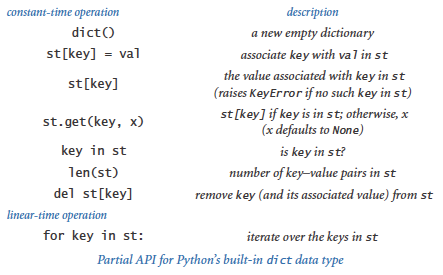The underlying implementation is a hash table, so ordered operations are not supported. As usual, since Python uses a lower-level language and does not impose on itself the overhead it imposes on all its users, that implementation will be more efficient and is preferred if ordered operations are not important.

As a simple example, the following `dict` client reads a sequence of strings from standard input, counts the number of times each string appears, and writes the strings and their frequencies. The strings do not come out in sorted order.

 ```import stdio st = dict() while not stdio.isEmpty(): word = stdio.readString() st[word] = 1 + st.get(word, 0) for word, frequency in st.iteritems(): stdio.writef('%s %4d\n', word, frequency) ```

Several examples of `dict` clients appear in the exercises at the end of this section.

## Set Data Type

As a final example, we consider a data type that is simpler than a symbol table, still broadly useful, and easy to implement with hashing or with BSTs. A set is a collection of distinct keys, like a symbol table with no values. For example, we could implement a set by deleting references to values in hashst.py or bst.py. Again, Python provides a `set` data type that is implemented in a lower-level language. This is a partial API: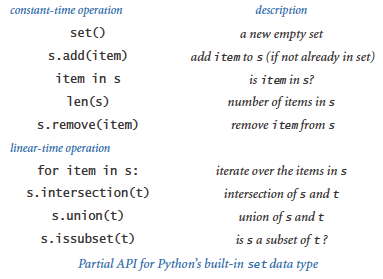For example, consider the task of reading a sequence of strings from standard input and writing the first occurrence of each string (thereby removing duplicates). We might use a `set`, as in the following client code:

 ```import stdio distinct = set() while not stdio.isEmpty(): key = stdio.readString() if key not in distinct: distinct.add(key) stdio.writeln(key) ```

You can find several other examples of set clients in the exercises at the end of this section.

Should you use Python's built-in `dict` and `set` data types? Of course, if they support the operations that you need, because they are written in a lower-level language, not subject to the overhead Python imposes on user code, and therefore are likely to be faster than anything that you could implement yourself. But if your application needs order-based operations like finding the minimum or maximum, you may wish to consider BSTs.

#### Q & A

Q. Can I use an array (or Python list) as a key in a `dict` or `set`?

A. No, the built-in `list` data type is mutable, so you should not use arrays as keys in a symbol table or set. In fact, Python lists are not hashable, so you cannot use them as keys in a `dict` or `set`. The built-in `tuple` data type is immutable (and hashable), so you can that instead.

Q. Why doesn't my user-defined data type work with `dict` or `set`?

A. By default, user-defined types are hashable, with `hash(x)` returning `id(x)` and `==` testing reference equality. While these default implementations satisfy the hashable requirements, they rarely provide the behavior you want.

Q. Why can't I return a Python list directly in the special method `__iter__()`? Why must I instead call the built-in `iter()` function with the Python list as an argument?

A. A Python list is an iterable object (because it has an `__iter__()` method that returns an iterator) but it is not an iterator.

Q. Which data structure does Python use to implement `dict` and `set`?

A. Python uses an open-addressing hash table, which is a cousin of the separate-chaining hash table we considered in this section. Python's implementation is highly optimized and written in a low-level programming language.

Q. Does Python provide language support for specifying `set` and `dict` objects?

A. Yes, you can specify a `set` by enclosing in curly braces a comma-separated list of its items. You can specify a `dict` by enclosing in curly braces a comma-separated list of its key'value pairs, with a colon between each key and its associated value.

 ```stopwords = {'and', 'at', 'of', 'or', on', 'the', 'to'} grades = {'A+':4.33, 'A':4.0, 'A-':3.67, 'B+':3.33, 'B':3.0} ```

Q. Does Python provide a built-in data type for an ordered symbol table (or ordered set) that supports ordered iteration, order statistics, and range search?

A. No. If you need only ordered iteration (with comparable keys), you could use Python's `dict` data type and sort the keys (and pay a performance hit for sorting). For example, if you use a `dict` instead of a binary search tree in index.py, you can arrange to write the keys in sorted order by using code like

 ```for word in sorted(st): ```

If you need other ordered symbol table operations (such as range search or order statistics), you can use our binary search tree implementation (and pay a performance hit for using a data type that is implemented in Python).

#### Exercises

1. Modify lookup.py to to make a program `lookupandput.py` that allows put operations to be specified on standard input. Use the convention that a plus sign indicates that the next two strings typed are the key-value pair to be inserted.

2. Modify lookup.py to make a program `lookupmultiple.py` that handles multiple values having the same key by putting the values in an array, as in index.py, and then writing them all out on a get request, as follows:

 ```% python lookupmultiple.py amino.csv 3 0 Leucine TTA TTG CTT CTC CTA CTG ```
3. Modify index.py to make a program `indexbykeyword.py` that takes a file name from the command line and makes an index from standard input using only the keywords in that file. Note: Using the same file for indexing and keywords should give the same result as index.py.

4. Modify index.py to make a program `indexlines.py` that considers only consecutive sequences of letters as keys (no punctuation or numbers) and uses line numbers instead of word position as the value. This functionality is useful for programs: when given a python program as input, `indexlines.py` should write an index showing each keyword or identifier in the program, along with the line numbers on which it occurs.

5. Develop an implementation `OrderedSymbolTable` of the symbol-table API that maintains parallel arrays of keys and values, keeping them in key-sorted order. Use binary search for get, and move larger elements to the right by one position for put (using resizing arrays to keep the array length linear in the number of key'value pairs in the table). Test your implementation with index.py, and validate the hypothesis that using such an implementation for index.py takes time proportional to the product of the number of strings and the number of distinct strings in the input.

6. Develop an implementation `LinkedSymbolTable` of the symbol-table API that maintains a linked list of nodes containing keys and values, keeping them in arbitrary order. Test your implementation with index.py, and validate the hypothesis that using such an implementation for index.py takes time proportional to the product of the number of strings and the number of distinct strings in the input.

7. Compute `hash(x) % 5` for the single-character keys

 ```E A S Y Q U E S T I O N ```

Draw the hash table created when the `i`th key in this sequence is associated with the value `i`, for `i` from 0 to 11.

8. What is wrong with the following `__hash__()` implementation?

 ```def __hash__(self): return -17 ```

Solution: While technically it satisfies the conditions needed for a data type to be hashable (if two objects are equal, they have the same hash value), it will lead to poor performance because we expect hash(x) % m to divide keys into m groups of roughly equal size.

9. Extend `Complex` (as defined in complex.py from Section 3.2) and `Vector` (as defined in vector.py from Section 3.3) to make them hashable by implementing the special methods `__hash__()` and `__eq__()`.

10. Modify hashst.py to use a resizing array so that the average length of the list associated with each hash value is between 1 and 8.

11. Draw all the different BSTs that can represent the key sequence

 ```best of it the time was ```
12. Draw the BST that results when you insert items with keys

 ```E A S Y Q U E S T I O N ```

in that order into an initially empty tree. What is the height of the resulting BST?

13. Suppose we have integer keys between 1 and 1000 in a BST and search for 363. Which of the following cannot be the sequence of keys examined?

 ```2 252 401 398 330 363 399 387 219 266 382 381 278 363 3 923 220 911 244 898 258 362 363 4 924 278 347 621 299 392 358 363 5 925 202 910 245 363 ```
14. Suppose that the following 31 keys appear (in some order) in a BST of height 5:

 ```10 15 18 21 23 24 30 31 38 41 42 45 50 55 59 60 61 63 71 77 78 83 84 85 86 88 91 92 93 94 98 ```

Draw the top three nodes of the tree (the root and its two children).

15. Describe the effect on performance if you replaced hashst.py with bst.py in lookup.py. To protect against the worst case, call `stdrandom.shuffle(database)` before populating the symbol table.

16. True or false: Given a BST, let x be a leaf node, and let p be its parent. Then either (i) the key of p is the smallest key in the BST larger than the key of x or (ii) the key of p is the largest key in the BST smaller than the key of x.

17. Modify the class `SymbolTable` in hashst.py to make a class `Set` that implements the constant-time operations in the partial API given in the text for Python's built-in `set` data type.

18. Modify the class `OrderedSymbolTable` in bst.py to make a class `OrderedSet` that implements the constant-time operations in the partial API given in the text for Python's built-in `set` data type, assuming that the keys are comparable.

19. Modify hashst.py to support the client code `del st[key]` by adding a method `__delitem__()` that takes a key argument and removes that key (and the corresponding value) from the symbol table, if it exists. Use a resizing array to ensure that the average length of the list associated with each hash value is between 1 and 8.

20. Implement `__str__()` for bst.py, using a recursive helper method. As usual, you can accept quadratic performance because of the cost of string concatenation. Extra credit: Compose a linear-time `__str__()` method for bst.py that uses an array and the `join()` method of Python's built-in `str` data type.

21. A concordance is an alphabetical list of the words in a text that gives all word positions where each word appears. Thus, `python index.py 0 0` produces a concordance. In a famous incident, one group of researchers tried to establish credibility while keeping details of the Dead Sea Scrolls secret from others by making public a concordance. Compose a program `invertconcordance.py` that takes a command-line argument `n`, reads a concordance from standard input, and writes the first `n` words of the corresponding text on standard output.

22. Run experiments to validate the claims in the text that the put operations and get requests for lookup.py are constant-time operations when using hashst.py with resizing arrays, as described in a previous exercise. Develop test clients that generate random keys and also run tests for various data sets, either from this booksite or of your own choosing.

23. Run experiments to validate the claims in the text that the put operations and get requests for index.py are logarithmic in the size of the symbol table when using bst.py. Develop test clients that generate random keys and also run tests for various data sets, either from this booksite or of your own choosing.

24. Modify bst.py to add methods `min()` and `max()` that return the smallest (or largest) key in the table (or `None` if the table is empty).

25. Modify bst.py to add methods `floor()` and `ceiling()` that take as an argument a key and return the largest (smallest) key in the set that is no larger (no smaller) than the given key.

26. Modify bst.py to support the special `len()` function by implementing a special method `__len__()` that returns the number of key'value pairs in the symbol table. Use the approach of storing within each `_Node` the number of nodes in the subtree rooted there.

27. Modify bst.py to add a method `rangeSearch()` that take two keys `lo` and `hi` as arguments and return an iterator over all keys that are between `lo` and `hi`. The running time should be proportional to the height plus the number of keys in the range.

28. Modify bst.py to add a method `rangeCount()` that takes keys as arguments and returns the number of keys in a BST between the two given keys. Your method should take time proportional to the height of the tree. Hint: First complete the previous exercise.

29. Modify bst.py to support the client code `del st[key]` by adding a method `__delitem__()` that takes a key argument and removes that key (and the corresponding value) from the symbol table, if it exists. Hint: This operation is more difficult than it might seem. Replace the key and its associated value with the next largest key in the BST and its associated value; then remove from the BST the node that contained the next largest key.

30. Modify the symbol-table API to handle values with duplicate keys by having `get()` return an iterator for the values having a given key. Reimplement hashst.py and bst.py as dictated by this API. Discuss the pros and cons of this approach versus the one given in the text.

31. Suppose that `a[]` is an array of hashable objects. What is the effect of the following statement?

 ```a = list(set(a)) ```
32. Recompose lookup.py and index.py using a `dict` instead of using hashst.py and bst.py, respectively. Compare performance.

33. Compose a `dict` client that creates a symbol table mapping letter grades to numerical scores, as in the table below, and then reads from standard input a list of letter grades and computes their average (GPA).

 ``` A+ A A- B+ B B- C+ C C- D F 4.33 4.00 3.67 3.33 3.00 2.67 2.33 2.00 1.67 1.00 0.00 ```
34. Implement the `buy()` and `sell()` methods in stockaccount.py (from Section 3.2). Use a `dict` to store the number of shares of each stock.

#### Binary Tree Exercises

The following exercises are intended to give you experience in working with binary trees that are not necessarily BSTs. They all assume a Node class with three instance variables: a positive double value and two Node references. As with linked lists, you will find it helpful to make drawings using the visual representation shown in the text.

1. Implement the following functions, each of which takes as an argument a `Node` that is the root of a binary tree.

• `size(node)`:number of nodes in the tree rooted at `node`
• `leaves(node)`: number of nodes in the tree rooted at `node` whose links are both `None`
• `total(node)`: sum of the key values in all nodes in the tree rooted at `node`

Your methods should all run in linear time.

2. Implement a linear-time function `height()` that returns the maximum number of nodes on any path from the root to a leaf node (the height of the empty tree is 0; the height of a one-node tree is 1).

3. A binary tree is heap-ordered if the key at the root is larger than the keys in all of its descendants. Implement a linear-time function `heapOrdered()` that returns `True` if the tree is heap-ordered, and `False` otherwise.

4. Given a binary tree, a single-value subtree is a maximal subtree that contains the same value. Design a linear-time algorithm that counts the number of single-value subtrees in a binary tree.

5. A binary tree is balanced if both its subtrees are balanced and the height of its two subtrees differ by at most 1. Implement a linear-time method `balanced()` that returns `True` if the tree is balanced, and `False` otherwise.

6. Two binary trees are isomorphic if only their key values differ (that is, they have the same shape). Implement a linear-time function `isomorphic()` that takes two tree references as arguments and returns `True` if they refer to isomorphic trees, and `False` otherwise. Then, implement a linear-time function `eq()` that takes two tree references as arguments and returns `True` if they refer to identical trees (isomorphic with the same key values), and `False` otherwise.

7. Compose a function `levelOrder()` that writes BST keys in level order: first write the root; then the nodes one level below the root, from left to right; then the nodes two levels below the root, from left to right; and so forth. Hint: Use a `Queue`.

8. Implement a linear-time function `isBST()` that returns `True` if the binary tree is a BST, and `False` otherwise.

Solution: This task is a bit more difficult than it might seem. Use a recursive helper function `_inRange()` that takes two additional arguments `lo` and `hi` and returns `True` if the binary tree is a BST and all its values are between `lo` and `hi`, and use `None` to represent both the smallest possible key and the largest possible key.

 ```def _inRange(node, lo, hi): if node is None: return True if (lo is not None) and (node.item <= lo): return False if (hi is not None) and (hi <= node.item): return False if not _inRange(node.left, lo, node.item): return False if not _inRange(node.right, node.item, hi): return False return True def _isBST(node): return _inRange(node, None, None) ```

We note that this implementation uses both the < and <= operators, whereas our binary search tree code uses only the < operator.

9. Compute the value returned by `mystery()` on some sample binary trees, and then formulate a hypothesis about the value and prove it.

 ```def mystery(node): if node is None: return 1 return mystery(node.left) + mystery(node.right) ```

#### Creative Exercises

1. Spell checking. Compose a `set` client `spellchecker.py` that takes as a command-line argument the name of a file containing a dictionary of words, and then reads strings from standard input and writes any string that is not in the dictionary. Use the file words.utf-8.txt. Extra credit: Augment your program to handle common suffixes such as -ing or -ed.

2. Spell correction. Compose a `dict` client `spellcorrector.py` that serves as a filter that replaces commonly misspelled words on standard input with a suggested replacement, writing the result to standard output. Take as a command-line argument a file that contains common misspellings and corrections. Use the file misspellings.txt, which contains many common misspellings.

3. Web filter. Compose a `set` client `webblocker.py` that takes as a command-line argument the name of a file containing a list of objectionable websites, and then reads strings from standard input and writes only those websites not on the list.

4. Set operations. Add the methods `union()` and `intersection()` to `OrderedSet` (see a previous exercise in this section), each of which takes two sets as arguments and that return the union and intersection, respectively, of those two sets.

5. Frequency symbol table. Develop a data type `FrequencyTable` that supports the following operations: `click()` and `count()`, both of which take string arguments. The data-type value is an integer that keeps track of the number of times the `click()` operation has been called with the given string as an argument. The `click()` operation increments the count by 1, and the `count()` operation returns the value, possibly 0. Clients of this data type might include a web traffic analyzer, a music player that counts the number of times each song has been played, phone software for counting calls, and so forth.

6. 1D range searching. Develop a data type that supports the following operations: insert a date, search for a date, and count the number of dates in the data structure that lie in a particular interval. Use Python's `datetime.Date` data type.

7. Non-overlapping interval search. Given a list of non-overlapping intervals of integers, compose a function that takes an integer argument and determines in which, if any, interval that value lies. For example, if the intervals are 1643-2033, 5532-7643, 8999-10332, and 5666653-5669321, then the query point 9122 lies in the third interval and 8122 lies in no interval.

8. IP lookup by country. Compose a `dict` client that uses the data file ip-by-country.csv to determine from which country a given IP address is coming. The data file has five fields: beginning of IP address range, end of IP address range, two-character country code, three-character country code, and country name. The IP addresses are non-overlapping. Such a database tool can be used for credit card fraud detection, spam filtering, auto-selection of language on a website, and web server log analysis.

9. Inverted index of web pages with single-word queries. Given a list of web pages, create a symbol table of words contained in the web pages. Associate with each word a list of web pages in which that word appears. Compose a program that reads in a list of web pages, creates the symbol table, and supports single-word queries by returning the list of web pages in which that query word appears.

10. Inverted index of web pages with multi-word queries. Extend the previous exercise so that it supports multi-word queries. In this case, output the list of web pages that contain at least one occurrence of each of the query words.

11. Multiple-word search (unordered). Compose a program that takes `k` keywords from the command line, reads in a sequence of words from standard input, and identifies the smallest interval of text that contains all of the `k` keywords (not necessarily in the same order). You do not need to consider partial words.

12. Multiple-word search (ordered). Repeat the previous exercise, but now assume the keywords must appear in the same order as specified.

13. Repetition draw in chess. In the game of chess, if a board position is repeated three times with the same side to move, the side to move can declare a draw. Describe how you could test this condition using a computer program.

14. Registrar scheduling. The registrar at a prominent Northeastern university recently scheduled an instructor to teach two different classes at the same exact time. Help the registrar prevent future mistakes by describing a method to check for such conflicts. For simplicity, assume all classes run for 50 minutes and start at 9, 10, 11, 1, 2, or 3.

15. Entropy. We define the relative entropy of a text corpus with n words, k of which are distinct as

E = 1 / (n lg n) (p0 lg(k/p0) + p1 lg(k/p1) + ... + pk-1 lg(k/pk-1))

where pi is the fraction of times that word i appears. Compose a program that reads in a text corpus and writes the relative entropy. Convert all letters to lowercase and treat punctuation marks as whitespace.

16. Order statistics. Add to bst.py a method `select()` that takes an integer argument `k` and returns the `k`th smallest key in the BST. Maintain subtree sizes in each node. The running time should be proportional to the height of the tree.

17. Rank query. Add to bst.py a method `rank()` that takes a key as an argument and returns the number of keys in the BST that are strictly smaller than key. Maintain subtree sizes in each node. The running time should be proportional to the height of the tree.

18. Random element. Add to bst.py a method `random()` that returns a random key. Maintain subtree sizes in each node. The running time should be proportional to the height of the tree.

19. Queue with no duplicates. Create a data type that is a queue, except that an element may appear on the queue at most once at any given time. Ignore requests to insert an item if it is already on the queue.

20. Unique substrings of a given length. Compose a program that reads in text from standard input and calculates the number of unique substrings of a given length `k` that it contains. For example, if the input is `CGCGGGCGCG`, then there are five unique substrings of length 3: `CGC`, `CGG`, `GCG`, `GGC`, and `GGG`. This calculation is useful in data compression. Hint: Use the string slice `s[i:i+k]` to extract the `i`th substring and insert into a symbol table. Test your program on the file pi-10million.txt, which contains the first 10 million digits of π.

21. Generalized queue. Implement a class that supports the following API: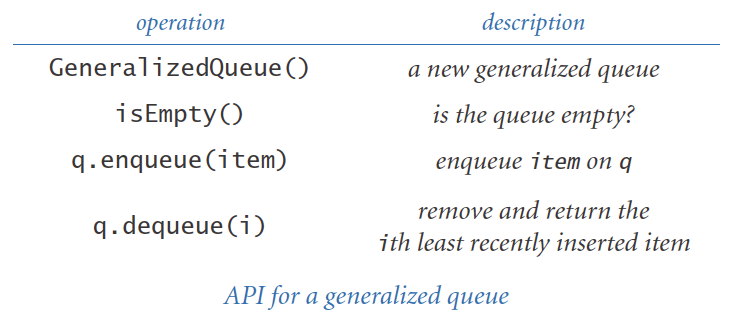Use a BST that associates the kth element inserted with the key k and maintains in each node the total number of nodes in the subtree rooted at that node. To find the ith least recently added item, search for the ith smallest element in the BST.

22. Dynamic discrete distribution. Create a data type that supports the following two operations: `add()` and `random()`. The `add()` method should insert a new item into the data structure if it has not been seen before; otherwise, it should increase its frequency count by 1. The `random()` method should return an element at random, where the probabilities are weighted by the frequency of each element. Use space proportional to the number of items.

23. Password checker. Compose a program that takes a string as a command-line argument and a dictionary of words from standard input, and checks whether the string is a 'good' password. Here, assume 'good' means that it (1) is at least eight characters long, (2) is not a word in the dictionary, (3) is not a word in the dictionary followed by a digit 0-9 (e.g., hello5), (4) is not two words in the dictionary concatenated together (e.g., helloworld), and (5) none of (2) through (4) hold for reverses of words in the dictionary. The file words.utf-8.txt contains a dictionary of words.

24. Random phone numbers. Compose a program that takes a command-line argument n and writes n random phone numbers of the form (xxx) xxx-xxxx. Use a `set` to avoid choosing the same number more than once. Use only legal area codes, as found in the file phone-na.csv.

25. Sparse vectors. An n-dimensional vector is sparse if its number of nonzero values is small. Your goal is to represent a vector with space proportional to its number of nonzeros, and to be able to add two sparse vectors in time proportional to the total number of nonzeros. Implement a class that supports the following API: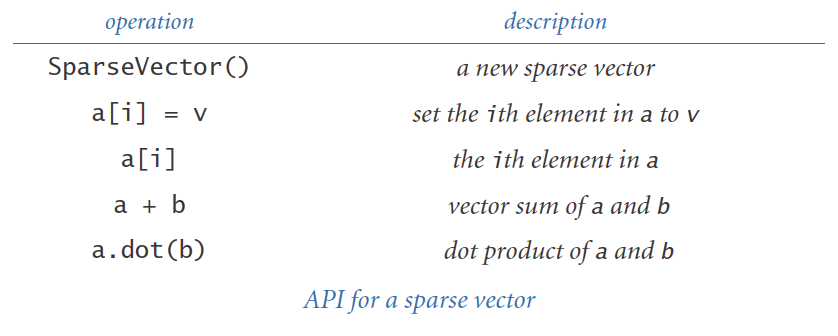26. Sparse matrices. An n-by-n matrix is sparse if its number of nonzeros is proportional to n (or less). Your goal is to represent a matrix with space proportional to n, and to be able to add and multiply two sparse matrices in time proportional to the total number of nonzeros (perhaps with an extra log n factor). Implement a class that supports the following API: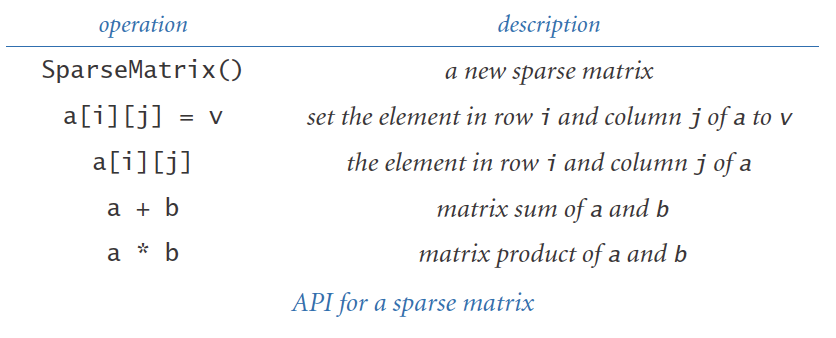27. Mutable string. Create a data type named `MutableString` that is the same as the Python `str` data type but is mutable. It should support these operations:

• `ms[i]`: return the `i`th character of `MutableString` object `ms`
• `ms[i] = c`: change the `i`th character of `MutableString` object `ms` to `c`.
• `ms.insert(i, c)`: insert the character `c` into `MutableString` object `ms` before index `i`.
• `del ms[i]`: delete the `i`th character of `MutableString` object `ms`

Use a BST to implement those operations in logarithmic time. Then compose other methods — a constructor, a `__str__()` method, comparison methods, a `__contains__()` method, an `__iter__()` method, and so forth — to make the data type reasonably complete.

28. Assignment statements. Compose a program to parse and evaluate programs consisting of assignment and write statements with fully parenthesized arithmetic expressions (see evaluate.py from Section 4.3). For example, given the input

 ```A = 5 B = 10 C = A + B D = C * C write(D) ```

your program should write the value 225. Assume that all variables and values are floats. Use a symbol table to keep track of variable names.

29. Codon usage table. Compose a program that uses a symbol table to write summary statistics for each codon in a genome taken from standard input (frequency per thousand), like the following:
 ```UUU 13.2 UCU 19.6 UAU 16.5 UGU 12.4 UUC 23.5 UCC 10.6 UAC 14.7 UGC 8.0 UUA 5.8 UCA 16.1 UAA 0.7 UGA 0.3 UUG 17.6 UCG 11.8 UAG 0.2 UGG 9.5 CUU 21.2 CCU 10.4 CAU 13.3 CGU 10.5 CUC 13.5 CCC 4.9 CAC 8.2 CGC 4.2 CUA 6.5 CCA 41.0 CAA 24.9 CGA 10.7 CUG 10.7 CCG 10.1 CAG 11.4 CGG 3.7 AUU 27.1 ACU 25.6 AAU 27.2 AGU 11.9 AUC 23.3 ACC 13.3 AAC 21.0 AGC 6.8 AUA 5.9 ACA 17.1 AAA 32.7 AGA 14.2 AUG 22.3 ACG 9.2 AAG 23.9 AGG 2.8 GUU 25.7 GCU 24.2 GAU 49.4 GGU 11.8 GUC 15.3 GCC 12.6 GAC 22.1 GGC 7.0 GUA 8.7 GCA 16.8 GAA 39.8 GGA 47.2 ```